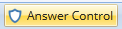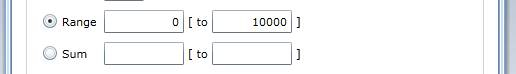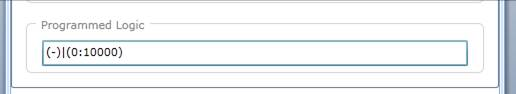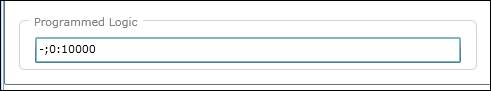# How to use range to ensure the quality of collected data - example 1

It cannot be overemphasized enough that the range and verify attributes are critical for ensuring quality data.

Example

You have the following question: “What was your income last month?” These are the following assumptions you will use:

• Question type will be numeric.
• A quantitative variable with a length of 5 digits would allow an income of \$ 99,999.
• Some respondents would not want to divulge their income. Hence, no answer IS allowed, hence the range no answer should be included (-).
• Let us say that nobody makes more than \$ 10,000. Range = 0:10000.
1. Open the answer control interface by clicking the answer control icon2. Specify the range control (From – to) The programmed logic will appear below3. in the “Programmed logic”, e.g. \Q1 (only the answers given in Question number 1)